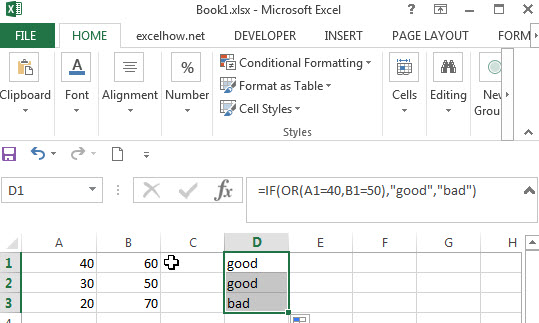# Excel IF formula with OR logical function

This post will guide you how to use IF function combining with OR logical function in Excel.

If you want to check if one of several conditions is met in your excel workbook, if the test is TRUE, then you can take certain action. You can use the IF function combining with OR function to construct a condition statement. If the test is FALSE, then take another action.

## Excel formula using IF & OR function

The syntax of OR function in excel is as follow:

`=OR(condition1,[condition2],...)`

The Excel OR function returns TRUE if any of the conditions are TRUE in logic test. Otherwise, it returns FALSE.

Now we want to check the results of two exam scores where the first score in cell A1 is greater than or equal to 40 OR the second score in cell B1 is greater than or equal to 50. If any of conditions are TRUE, then returns the text of “good”, and if FALSE returns “bad”.

Based on the above logic, we can write down the below IF formula:

`=IF(OR(A1=”40”,B1=”50”),”good”, ”bad”)`

We can enter the above formula into cell D1, and then drag the Fill Handle to the range D1:D3.it will apply for the other cells for this formula.So the difference from the IF&AND formula discussed in the previous post is that the IF&OR formula will return TRUE if any of conditions are true.

In the above formula, we just want to check both A1 and B1 cells, the Excel will take an action when the result of test condition is TRUE. In this case, we will add “good” text in the D1 cell. If the test condition is FALSE, assuming that you do not want to display anything in the cell D1, then we can write down the following excel if formula:

`=IF(OR(A1=”40”,B1=”50”),”good”,””)`

Normally, you can use as many AND/OR logical functions as your logic requires, but there is limitation for argument numbers, we can follow the below limitation for excel formula:

1. No more than 255 arguments in Microsoft excel 2016,2013,2010 and 2007
2. No more than 8192 characters in Microsoft excel 2016,2013,2010 and 2007
3. No more than 30 arguments and the length of formula should not exceed 1024 characters in Microsoft excel 2003 or lower version.

### Related Functions

• Excel OR function
The Excel OR function returns TRUE if any of the conditions are TRUE in logic test. Otherwise, it returns FALSE.
• Excel AND function
The Excel AND function returns TRUE if all of arguments are TRUE, and it returns FALSE if any of arguments are FALSE.The syntax of the AND function is as below:= AND (condition1,[condition2],…) …
• Excel IF function
The Excel IF function perform a logical test to return one value if the condition is TRUE and return another value if the condition is FALSE….
• Excel nested if function
The nested IF function is formed by multiple if statements within one Excel if function. This excel nested if statement makes it possible for a single formula to take multiple actions…

Related Posts

Extract matching values From Two Lists

Suppose that you are working with two lists containing few values, and you want to extract the matching values from those two lists into another separate list. You might prefer to manually extract the matching values from the two lists, ...

Extract Multiple Match Values into Separate Columns

If you have a few values/items in the excel sheet and you are thinking that with the aid of the “VlOOKUP” function you can look for a specific value, extract it and then put the matching item into the separate ...

VLOOKUP Formula | Faster Trick with 2 VLOOKUPS

This post will guide you how to use 2 VLOOKUPS function to looking up data entries from a given range of cells in Microsoft Excel. VLOOKUP with 2 lookups can be faster than a single VLOOKUP in certain scenarios. The ...

Extract all the matches with helper Column

With Excel's powerful functions IF, INDEX, and MATCH, we can find exactly what you're looking for with a few clicks of the mouse. This step-by-step tutorial will show how easy it is to extract data using these tools and more! ...

If Cell is This Value or That Value

IF function is frequently used in Excel worksheet to return you expect “true value” or “false value” based on the result of logical test. If you want to see if a cell is A or B, and if one of ...

If Value is Greater Than A Certain Value

IF function is frequently used in Excel worksheet to return you expect “true value” or “false value” based on the logical test result. If you want to see if a value in one cell is greater than a specific value, ...

If Cell is Not Blank

IF function is frequently used in Excel worksheet to return you expect “true value” or “false value” based on the result of created logical test. If you want to see if a cell is blank or not, and leave some ...

If Cell is Blank

IF function is frequently used in Excel worksheet to return you expect “true value” or “false value” based on the result of created logical test. If you want to see if a cell is blank or not, and leave some ...

If Cell Equals Certain Text String

IF function is frequently used in Excel worksheet to return you expect “true value” or “false value” based on the result of created logical test. If you want to see if cell equals a certain text string like “Win”, you ...

If Cell Contains Either Text1 or Text2

IF function is frequently used in Excel worksheet to return “true value” or “false value” based on the logical test result. If you want to see if cell contains certain substring1 like “abc” or substring2 like “def”, and returns true ...

Sidebar